# Electronics and Communication Engineering - Exam Questions Papers

31.

The logic circuit given below converts a binary code y1 y2, y3 into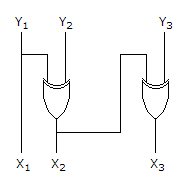A. Excess - 3 code B. Gray code C. BCD code D. Hamming

Explanation:

Let y1 = 1, y2 = 0, y3 = 1

then x1 = 1

x2 = y1y2 ⇒ 1 ⊕ 0 ⇒ 1

x3 = x2y3 = 1 ⊕ 0 = 1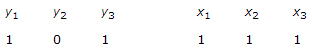which is gray code conversion.

32.

A system described by the following differential equation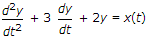is initially at rest. For input x(t) - 2u(t), the output y(t) is :

 A. (1 - 2e-t + e -2t) B. (1 + 2e-t - 2e -2t)u(t) C. (0.5 + e-t + 1.5e -2t)u(t) D. (0.5 + 2e-t + 2e -2t)u(t)

Explanation: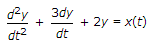Taking L.T.

s2y(s) + 3sy(s) + 2y(s) = x(s)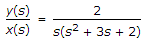I.L.T = (1 - 2e-t + e-2t)U(t).

33.

A transmission line terminates in two branches, each of length λ/4, as shown. The branches are terminated by 50Ω loads. The lines are lossless and have the characteristics impedances shown. Determine the impedance Z1 as seen by the source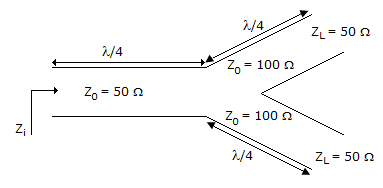A. 200Ω B. 100Ω C. 50Ω D. 25Ω

Explanation: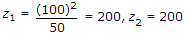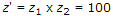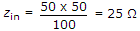.

34.

The Nyquist plot of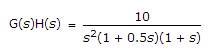A. will start from (ω = ∞) in the first quadrant and will terminate (ω = 0) in the second quadrant B. will start from (ω = ∞) in the fourth quadrant and will terminate (ω = 0) in the second quadrant C. will start form (ω = ∞) in the second quadrant and will terminate (ω = 0) in the fourth quadrant D. will start from (ω = ∞) in the first quadrant and will terminate (ω = 0) in the fourth quadrant

Explanation: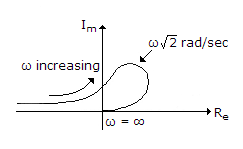35.

The counter shown in figure is built using 4 negative edge triggered toggle FFs. The FFs can be set synchronously when R = 0. The combinational logic required to realize a modulo 13 counter is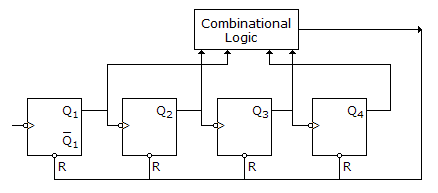A. F= Q4 Q3 Q2 Q1 B. F= Q4 + Q3 + Q2 + Q1 C. F = Q4 + Q3 + Q2 + Q1 D. F = Q4 Q3 Q2 Q1

Explanation:

According to figure, output of combinational logic is applied to R of all FF

N = 13 = (1101)2

The logic required is Q4 Q3 Q2 Q1 The gate used is a NAND.

Y = Q4 + Q3 + Q2 + Q1 .

#### Current Affairs 2022

Interview Questions and Answers